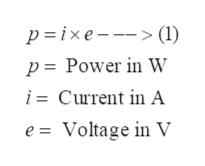# Find the power delivered to a resistor at t=3 ms if the current entering the resistor is i=5 cos (180t) A and the voltage across the resistors is e=3i V.

Question
1 views

Find the power delivered to a resistor at t=3 ms if the current entering the resistor is i=5 cos (180t) A and the voltage across the resistors is e=3i V.

check_circle

Step 1

Power delivered to a resistor can be defined as the product of the current flowing through the resistor and the voltage drop measured across the resistor. This is expressed by equation (1).help_outlineImage Transcriptionclosep ixe-- (1) p = Power in W i= Current in A e = Voltage in V fullscreen
Step 2

In this case, the current flowing through the resistor and voltage drop across the resistor are expressed by equation (2) and (3) respectively.

Step 3

Therefore, from equation (1), the power ...

### Want to see the full answer?

See Solution

#### Want to see this answer and more?

Solutions are written by subject experts who are available 24/7. Questions are typically answered within 1 hour.*

See Solution
*Response times may vary by subject and question.
Tagged in